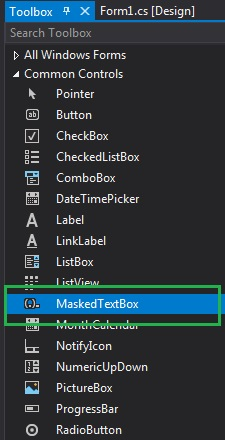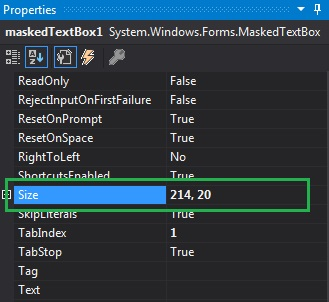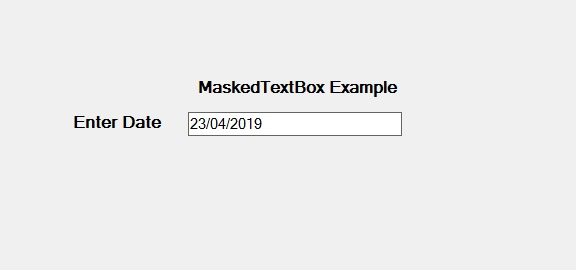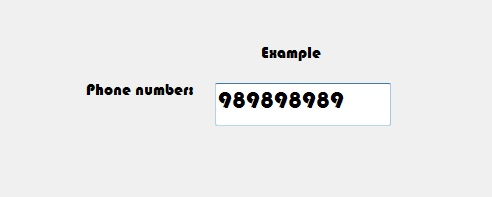Open In App

# How to set the size of the MaskedTextBox in C#?

In C#, MaskedTextBox control gives a validation procedure for the user input on the form like date, phone numbers, etc. Or in other words, it is used to provide a mask which differentiates between proper and improper user input. In MaskedTextBox control, you can set the size of the MaskedTextBox on the form using Size Property. This property represents both the height and width of the MaskedTextBox control in pixels. You can set this property in two different ways:

1. Design-Time: It is the easiest way to set the size of the MaskedTextBox as shown in the following steps:

• Step 1: Create a windows form as shown in the below image:
Visual Studio -> File -> New -> Project -> WindowsFormApp• Step 2: Next, drag and drop the MaskedTextBox control from the toolbox on the form as shown in the below screenshot.• Step 3: After drag and drop you will go to the properties of the MaskedTextBox and set the size of the MaskedTextBox as shown in the below image:Output:2. Run-Time: It is a little bit trickier than the above method. In this method, you can set the size of the MaskedTextBox control programmatically with the help of given syntax:

`public System.Drawing.Size Size { get; set; }`

Here, Size represents the height and width of the MaskedTextBox control in pixels. The following steps show how to set the size of the MaskedTextBox dynamically:

```// Creating a MaskedTextBox
```
• Step 2: After creating MaskedTextBox, set the Size property of the MaskedTextBox provided by the MaskedTextBox class.
```// Setting the Size
m.Size = new Size(176, 20);
```
• Step 3: And last add this MaskedTextBox control to the form using the following statement:
```// Adding MaskedTextBox control on the form
 `using` `System;``using` `System.Collections.Generic;``using` `System.ComponentModel;``using` `System.Data;``using` `System.Drawing;``using` `System.Linq;``using` `System.Text;``using` `System.Threading.Tasks;``using` `System.Windows.Forms;`` ` `namespace` `WindowsFormsApp36 {`` ` `public` `partial` `class` `Form1 : Form {`` ` `    ``public` `Form1()``    ``{``        ``InitializeComponent();``    ``}`` ` `    ``private` `void` `Form1_Load(``object` `sender, EventArgs e)``    ``{`` ` `        ``// Creating and setting the ``        ``// properties of Label``        ``Label l1 = ``new` `Label();``        ``l1.Location = ``new` `Point(413, 98);``        ``l1.Size = ``new` `Size(176, 20);``        ``l1.Text = ``" Example"``;``        ``l1.Font = ``new` `Font(``"Bauhaus 93"``, 12);`` ` `        ``// Adding label on the form``        ``this``.Controls.Add(l1);`` ` `        ``// Creating and setting ``        ``// the properties of Label``        ``Label l2 = ``new` `Label();``        ``l2.Location = ``new` `Point(242, 135);``        ``l2.Size = ``new` `Size(126, 20);``        ``l2.Text = ``"Phone number:"``;``        ``l2.Font = ``new` `Font(``"Bauhaus 93"``, 12);`` ` `        ``// Adding label on the form``        ``this``.Controls.Add(l2);`` ` `        ``// Creating and setting the ``        ``// properties of MaskedTextBox``        ``MaskedTextBox m = ``new` `MaskedTextBox();``        ``m.Location = ``new` `Point(374, 137);``        ``m.Mask = ``"000000000"``;``        ``m.Size = ``new` `Size(176, 20);``        ``m.Name = ``"MyBox"``;``        ``m.Font = ``new` `Font(``"Bauhaus 93"``, 18);`` ` `        ``// Adding MaskedTextBox ``        ``// control on the form``        ``this``.Controls.Add(m);``    ``}``}``}`